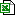Vostok ice core data (student spreadsheet)Vostok ice core data (student spreadsheet)
An Excel spreadsheet containing multiple lines of data from the Vostok ice core. Students will use these data to calculate paleotemperature, and the spreadsheet will automatically plot the results as a graph.

File 51452 is a 126kB Excel 2007 (.xlsx)